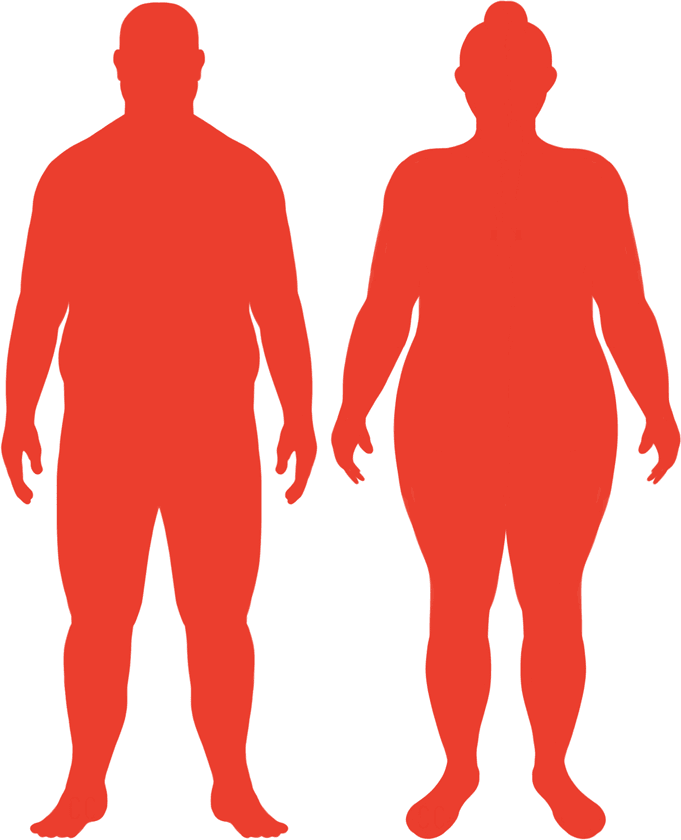# BMI Calculator

Result for 7'9" and 433 lbs.
Change Here.35.19

### Obese BMI

At 7'9" and 433 Pounds your BMI is 35.19

### BMI Scale

 Under Normal Over Obese
-64 lbs
to reach an
overweight bmi classification.
##### Overweight BMI Ends:
369.1 lbs
-126 lbs
to reach a
normal bmi classification.
307.5 lbs

### BMI Grading Table2 for Height 7'9"

 Weight Weight Class Below 227.6 lbs Underweight 227.6 lbs - 307.5 lbs Normal Weight 307.5 lbs - 369.1 lbs Overweight 369.1 lbs and Above Obese

### Weight Loss

If you were seeking a normal BMI weight of 307.5lbs, and you lost two pounds a week4, then you could reach a normal BMI in
63 Weeks
Or to be considered overweight, a goal weight of 369.1 lbs could be reached in
32 Weeks

### Obese BMI Silhouette### Ideal Weight for a 7 foot 9 Male or Female2

The ideal weight range using the BMI for a male or female with a height of 7'9" is

### Between

227.6 lbs

and
307.5 lbs
How to Calculate BMI3 for 7'9" and 433 lbs
(7 x 12) + 9 = 93
(433 / (93 x 93)) x 703
= 35.194704590126
7 = foot | 9 = inch | 433 = weight lbs

### Conversion

• 7 foot 9 = 93 inches
• 7 foot 9 = 236.22cm
• 433 pounds = 30 stone 13 pounds
• 433 pounds = 196.41 kg
• 433 pounds = 196405.5 grams

### 7'9" and 433 lbs Summary

What is the BMI for a 7'9" and 433 lbs female? 35.19 BMI, Obese.
What is the BMI for a 7'9" and 433 lbs male? 35.19 BMI, Obese.
What is the ideal weight for a 7'9" female? Between: 227.6lbs and 307.5lbs
What is the ideal weight for a 7'9" male? Between: 227.6lbs and 307.5lbs
If I am 7ft 9in and weigh 433 lbs, is that a good weight for my height? Under the BMI classification, 433 lbs is classed as being Obese.